# Logic Gates in Python

A Logic gate is an elementary building block of any digital circuits. It takes one or two inputs and produces output based on those inputs. Outputs may be high (1) or low (0). Logic gates are implemented using diodes or transistors. It can also be constructed using vacuum tubes, electromagnetic elements like optics, molecule etc. In a computer, most of the electronic circuits are made up logic gates. Logic gates are used to create a circuit that performs calculations, data storage or shows off object-oriented programming especially the power of inheritance.
There are seven basic logic gates defined, these are: AND gate, OR gate, NOT gate, NAND gate, NOR gate , XOR gate and XNOR gate.
1. AND Gate
The AND gate gives an output of 1 if both the two inputs are 1, it gives 0 otherwise.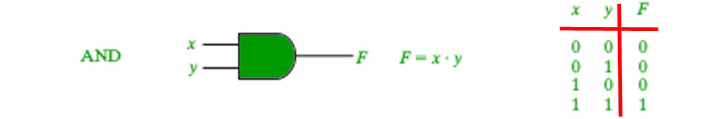`# Python3 program to illustrate ` `# working of AND gate ` ` `  `def` `AND (a, b): ` ` `  `    ``if` `a ``=``=` `1` `and` `b ``=``=` `1``: ` `        ``return` `True` `    ``else``: ` `        ``return` `False` ` `  `# Driver code ` `if` `__name__``=``=``'__main__'``: ` `    ``print``(AND(``1``, ``1``)) ` ` `  `    ``print``(``"+---------------+----------------+"``) ` `    ``print``(``" | AND Truth Table | Result |"``) ` `    ``print``(``" A = False, B = False | A AND B ="``,AND(``False``,``False``),``" | "``) ` `    ``print``(``" A = False, B = True | A AND B ="``,AND(``False``,``True``),``" | "``) ` `    ``print``(``" A = True, B = False | A AND B ="``,AND(``True``,``False``),``" | "``) ` `    ``print``(``" A = True, B = True | A AND B ="``,AND(``True``,``True``),``" | "``) `

Output:

```True
+---------------+----------------
| AND Truth Table |    Result |
A = False, B = False | A AND B = False  |
A = False, B = True  | A AND B = False  |
A = True, B = False  | A AND B = False  |
A = True, B = True   | A AND B = True   |
```

2. NAND Gate
The NAND gate (negated AND) gives an output of 0 if both inputs are 1, it gives 1 otherwise.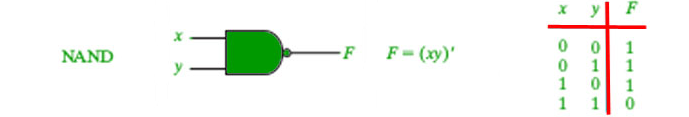`# Python3 program to illustrate ` `# working of NAND gate ` ` `  `def` `NAND (a, b): ` `    ``if` `a ``=``=` `1` `and` `b ``=``=` `1``: ` `        ``return` `False` `    ``else``: ` `        ``return` `True` ` `  `# Driver code ` `if` `__name__``=``=``'__main__'``: ` `    ``print``(NAND(``1``, ``0``)) ` ` `  `    ``print``(``"+---------------+----------------+"``) ` `    ``print``(``" | NAND Truth Table | Result |"``) ` `    ``print``(``" A = False, B = False | A AND B ="``,NAND(``False``,``False``),``" | "``) ` `    ``print``(``" A = False, B = True | A AND B ="``,NAND(``False``,``True``),``" | "``) ` `    ``print``(``" A = True, B = False | A AND B ="``,NAND(``True``,``False``),``" | "``) ` `    ``print``(``" A = True, B = True | A AND B ="``,NAND(``True``,``True``),``" | "``) `

Output:

```True
+---------------+----------------
| NAND Truth Table |    Result |
A = False, B = False | A AND B = True  |
A = False, B = True  | A AND B = True  |
A = True, B = False  | A AND B = True  |
A = True, B = True   | A AND B = False |
```

3. OR Gate
The OR gate gives an output of 1 if either of the two inputs are 1, it gives 0 otherwise.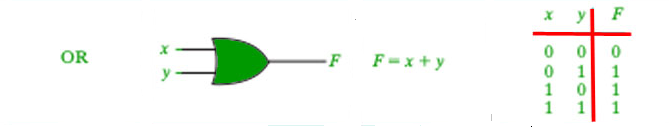`# Python3 program to illustrate ` `# working of OR gate ` ` `  `def` `OR(a, b): ` `    ``if` `a ``=``=` `1``: ` `        ``return` `True` `    ``elif` `b ``=``=` `1``: ` `        ``return` `True` `    ``else``: ` `        ``return` `False` ` `  `# Driver code ` `if` `__name__``=``=``'__main__'``: ` `    ``print``(OR(``0``, ``0``)) ` ` `  `    ``print``(``"+---------------+----------------+"``) ` `    ``print``(``" | OR Truth Table | Result |"``) ` `    ``print``(``" A = False, B = False | A AND B ="``,OR(``False``,``False``),``" | "``) ` `    ``print``(``" A = False, B = True | A AND B ="``,OR(``False``,``True``),``" | "``) ` `    ``print``(``" A = True, B = False | A AND B ="``,OR(``True``,``False``),``" | "``) ` `    ``print``(``" A = True, B = True | A AND B ="``,OR(``True``,``True``),``" | "``) `

Output:

```False
+---------------+----------------+
| OR Truth Table |    Result |
A = False, B = False | A AND B = False  |
A = False, B = True  | A AND B = True   |
A = True, B = False  | A AND B = True   |
A = True, B = True   | A AND B = True   |
```

4. XOR Gate
The XOR gate gives an output of 1 if either both inputs are different, it gives 0 if they are same.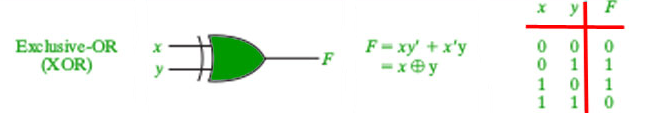`# Python3 program to illustrate ` `# working of Xor gate ` ` `  `def` `XOR (a, b): ` `    ``if` `a !``=` `b: ` `        ``return` `1` `    ``else``: ` `        ``return` `0` ` `  `# Driver code ` `if` `__name__``=``=``'__main__'``: ` `    ``print``(XOR(``5``, ``5``)) ` ` `  `    ``print``(``"+---------------+----------------+"``) ` `    ``print``(``" | XOR Truth Table | Result |"``) ` `    ``print``(``" A = False, B = False | A AND B ="``,XOR(``False``,``False``),``" | "``) ` `    ``print``(``" A = False, B = True | A AND B ="``,XOR(``False``,``True``),``" | "``) ` `    ``print``(``" A = True, B = False | A AND B ="``,XOR(``True``,``False``),``" | "``) ` `    ``print``(``" A = True, B = True | A AND B ="``,XOR(``True``,``True``),``" | "``) `

Output:

```0
+---------------+----------------+
| XOR Truth Table | Result |
A = False, B = False | A AND B = 0  |
A = False, B = True  | A AND B = 1  |
A = True, B = False  | A AND B = 1  |
A = True, B = True   | A AND B = 0  |
```

5. NOT Gate
It acts as an inverter. It takes only one input. If the input is given as 1, it will invert the result as 0 and vice-versa.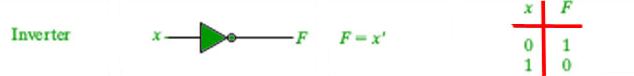`# Python3 program to illustrate ` `# working of Not gate ` ` `  `def` `NOT(a): ` `    ``if``(a ``=``=` `0``): ` `        ``return` `1` `    ``elif``(a ``=``=` `1``): ` `        ``return` `0` `# Driver code ` `if` `__name__``=``=``'__main__'``: ` `    ``print``(NOT(``0``)) ` ` `  `    ``print``(``"+---------------+----------------+"``) ` `    ``print``(``" | NOT Truth Table | Result |"``) ` `    ``print``(``" A = False | A NOT ="``,NOT(``False``),``" | "``) ` `    ``print``(``" A = True, | A NOT ="``,NOT(``True``),``" | "``) ` `  `

Output:

```1
+---------------+----------------+
| NOT Truth Table | Result |
A = False | A NOT = 1  |
A = True, | A NOT = 0  |
```

6. NOR Gate
The NOR gate (negated OR) gives an output of 1 if both inputs are 0, it gives 1 otherwise.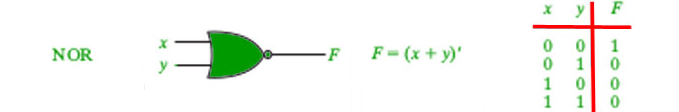`# Python3 program to illustrate ` `# working of NOR gate ` ` `  `def` `NOR(a, b): ` `    ``if``(a ``=``=` `0``) ``and` `(b ``=``=` `0``): ` `        ``return` `1` `    ``elif``(a ``=``=` `0``) ``and` `(b ``=``=` `1``): ` `        ``return` `0` `    ``elif``(a ``=``=` `1``) ``and` `(b ``=``=` `0``): ` `        ``return` `0` `    ``elif``(a ``=``=` `1``) ``and` `(b ``=``=` `1``): ` `        ``return` `0` ` `  `# Driver code  ` `if` `__name__``=``=``'__main__'``: ` `    ``print``(NOR(``0``, ``0``)) ` ` `  `    ``print``(``"+---------------+----------------+"``) ` `    ``print``(``" | NOR Truth Table | Result |"``) ` `    ``print``(``" A = False, B = False | A AND B ="``,NOR(``False``,``False``),``" | "``) ` `    ``print``(``" A = False, B = True | A AND B ="``,NOR(``False``,``True``),``" | "``) ` `    ``print``(``" A = True, B = False | A AND B ="``,NOR(``True``,``False``),``" | "``) ` `    ``print``(``" A = True, B = True | A AND B ="``,NOR(``True``,``True``),``" | "``) `

Output:

```1
+---------------+----------------+
| NOR Truth Table |   Result |
A = False, B = False | A AND B = 1  |
A = False, B = True  | A AND B = 0  |
A = True, B = False  | A AND B = 0  |
A = True, B = True   | A AND B = 0  |
```

7. XNOR Gate
The XNOR gate (negated XOR) gives an output of 1 both inputs are same and 0 if both are different.`# Python3 program to illustrate ` `# working of Not gate ` ` `  `def` `XNOR(a,b): ` `    ``if``(a ``=``=` `b): ` `        ``return` `1` `    ``else``: ` `        ``return` `0` `# Driver code ` `if` `__name__``=``=``'__main__'``: ` `    ``print``(XNOR(``1``,``1``)) ` ` `  `    ``print``(``"+---------------+----------------+"``) ` `    ``print``(``" | XNOR Truth Table | Result |"``) ` `    ``print``(``" A = False, B = False | A AND B ="``,XNOR(``False``,``False``),``" | "``) ` `    ``print``(``" A = False, B = True | A AND B ="``,XNOR(``False``,``True``),``" | "``) ` `    ``print``(``" A = True, B = False | A AND B ="``,XNOR(``True``,``False``),``" | "``) ` `    ``print``(``" A = True, B = True | A AND B ="``,XNOR(``True``,``True``),``" | "``) `

Output:

```1
+---------------+----------------+
| XNOR Truth Table |  Result |
A = False, B = False | A AND B = 1  |
A = False, B = True  | A AND B = 0  |
A = True, B = False  | A AND B = 0  |
A = True, B = True   | A AND B = 1  |
```

My Personal Notes arrow_drop_upCheck out this Author's contributed articles.

If you like GeeksforGeeks and would like to contribute, you can also write an article using contribute.geeksforgeeks.org or mail your article to contribute@geeksforgeeks.org. See your article appearing on the GeeksforGeeks main page and help other Geeks.

Please Improve this article if you find anything incorrect by clicking on the "Improve Article" button below.

Improved By : Akanksha_Rai

Article Tags :

3

Please write to us at contribute@geeksforgeeks.org to report any issue with the above content.